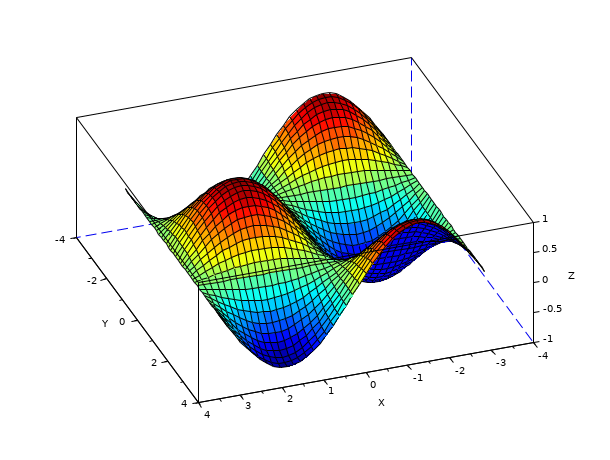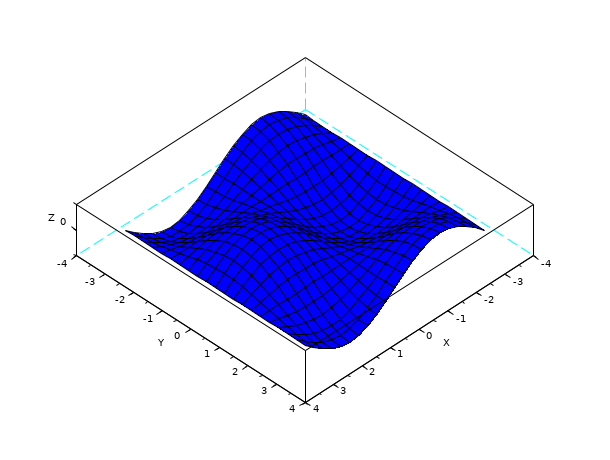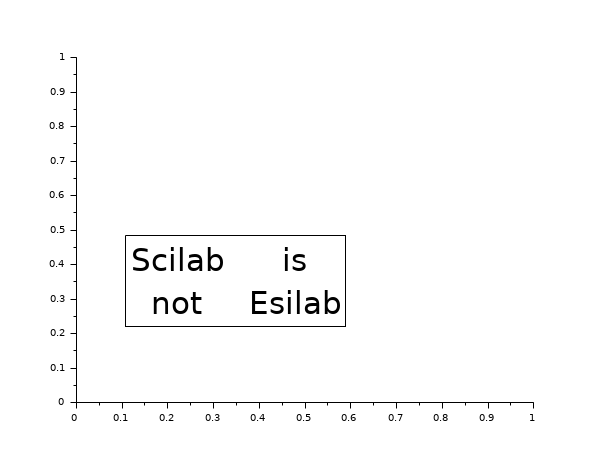Change language to:
Français - 日本語 - Português - Русский

See the recommended documentation of this function

Scilab Help >> Graphics > Graphics: Getting started

# Graphics: Getting started

graphics library overview

### 2d plotting

plot2d

Plot a curve

Example:plot2d2

Plot a curve as step function

Example:plot2d3

Plot a curve with vertical bars

Example:plot2d4

Plot a curve with arrows

Example:fplot2d

Plot a curve defined by a function

Example:champ

2D vector field

Example:fchamp

Direction field of a 2D first order ODE

Example:contour2d

Level curves of a surface on a 2D plot

grayplot

2D plot of a surface using colors

Example:fgrayplot

2D plot of a surface defined by a function using colors

Example:Sgrayplot

Smooth 2D plot of a surface using colors

Example:Sfgrayplot

Smooth 2D plot of a surface defined by a function using colors

Example:xgrid

Add a grid on a 2D plot

Example:errbar

Add vertical error bars on a 2D plot

Example:histplot

Plot a histogram

Example:Matplot

2D plot of a matrix using colors

Example:### 3d plotting

plot3d

Plot a surface

Example:plot3d1

Plot a surface with gray or color level

Example:fplot3d

Plot a surface defined by a function

Example:fplot3d1

Plot a surface defined by a function with gray or color level

Example:param3d

Plot one curve

Example:param3d1

Plots curves

Example:contour

Level curves on a 3D surface

Example:bar3d

3D representation of a bar diagram

Example:genfac3d

Compute facets of a 3D surface

Example:eval3dp

Compute facets of a 3D surface

Example:geom3d

Projection from 3D on 2D after a 3D plot

Example:### Line and polygon plotting

xpoly

Draw a polyline or a polygon

xpolys

Draw a set of polylines or polygons

xrpoly

Draw a regular polygon

xsegs

Draw unconnected segments

xfpoly

Fill a polygon

xfpolys

Fill a set of polygons

### Rectangle plotting

xrect

Draw a rectangle

xfrect

Fill a rectangle

xrects

Draw or fill a set of rectangles

### Arc plotting

xarc

Draw a part of an ellipse

xarcs

Draw parts of a set of ellipses

xfarc

Fill a part of an ellipse

xfarcs

Fill parts of a set of ellipses

### Arrow plotting

xarrows

Draw a set of arrows

### Strings

xstring

Draw strings

xstringl

Compute a box which surrounds strings

xstringb

Draw strings into a box

Example:xtitle

Add titles on a graphics window

Example:titlepage

Add a title in the middle of a graphics window

### Frames and axes

drawaxis

Draw an axis

plotframe

Plot a frame with scaling and gridsThis function is obsolete.

### Coordinates transformations

isoview

Set isometric plotting scales

scaling

Affine transformation of a set of points

rotate

Rotation of a set of points

xsetech

Set the sub-window of a graphics window for plotting

subplot

Divide a graphics window into a matrix of sub-windows

xchange

Transform real to pixel coordinates

### Colors

colormap

Using colormaps

getcolor

Dialog to select colors in the current colormap

Add new colors to the current colormap

graycolormap

Linear gray colormap

hotcolormap

Red to yellow colormap

### Graphics context

set

Set values of the graphics context

get

Retrieve the value of a property from the handle of a graphic or of an interactive object.

xlfont

xsave

Save graphics into a file

xs2bmp

Export graphics using the BMP format

xs2emf

Export graphics using the EMF formatOnly under Windows.

xs2eps

Export graphics using the EPS format

xs2gif

Export graphics using the GIF format

xs2jpg

Export graphics using the JPG format

xs2pdf

Export graphics using the PDF format

xs2png

Export graphics using the PNG format

xs2ppm

Export graphics using the PPM format

xs2ps

Export graphics using the PS format

xs2svg

Export graphics using the SVG format

### Graphics primitives

clf

Clear or reset the current graphic figure (window) to default values

driver

Select a graphics driver

xinit

Initialisation of a graphics driver

xend

Close a graphics session

replot

Redraw the current graphics window with new boundaries

show_window

Raise the current graphics window

xdel

Delete a graphics window

winsid

Return the list of graphics windows

xname

Change the name of the current graphics window

### Mouse position

xclick

Wait for a mouse click or an event in a graphic window

locate

Mouse selection of a set of points

xgetmouse

Get the mouse events and current position

### Interactive editor

edit_curv

Interactive graphics curve editor

### Graphics functions for automatic control

bode

Bode plot

Example:gainplot

Magnitude plot

Example:nyquist

Nyquist plot

Example:hallchart

Hall chart

Example:nicholschart

Nichols chart

Example:black

Black's diagram

Example:evans

Evans root locus

Example:sgrid

s-plane grid lines

Example:plzr

pole-zero plot

Example:zgrid

zgrid plot

Example:Report an issue << GlobalProperty Graphics Compound properties >>